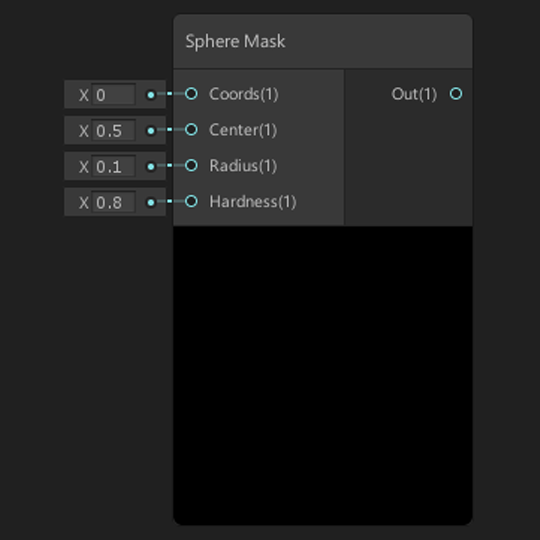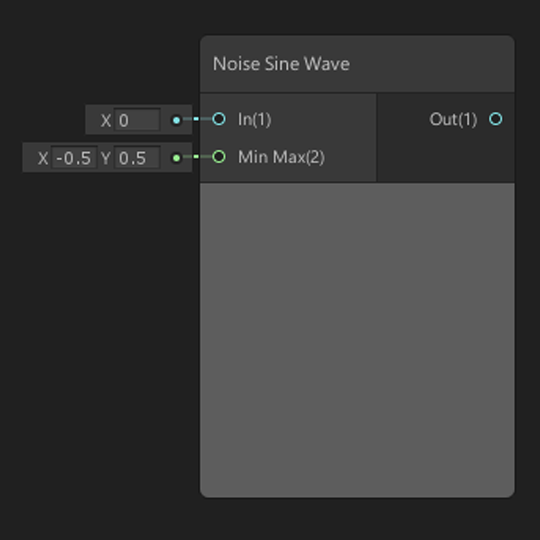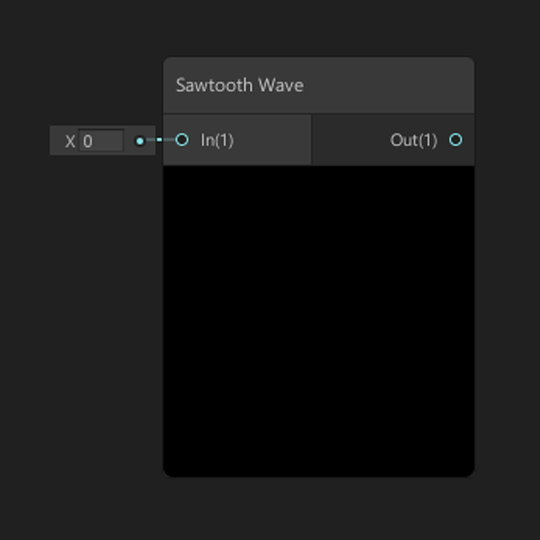# Math Nodes

Absolute Exponential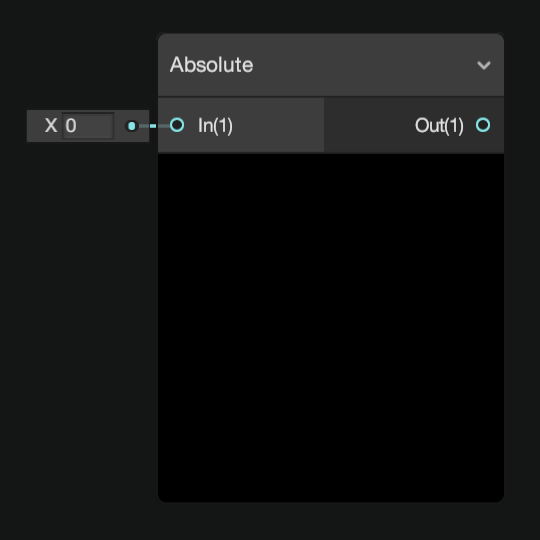Returns the absolute value of input In. Returns the exponential value of input In.
Length Log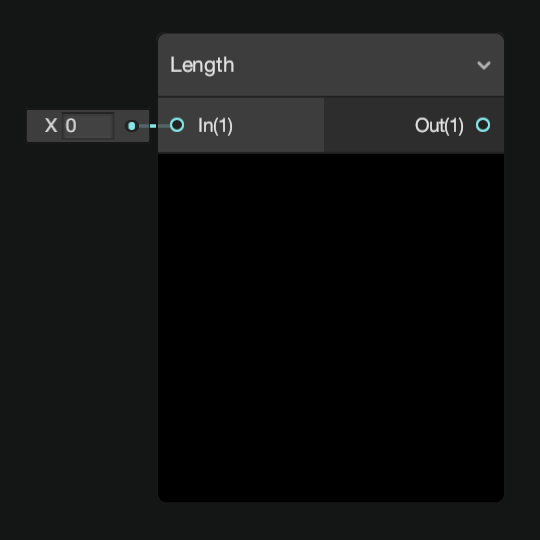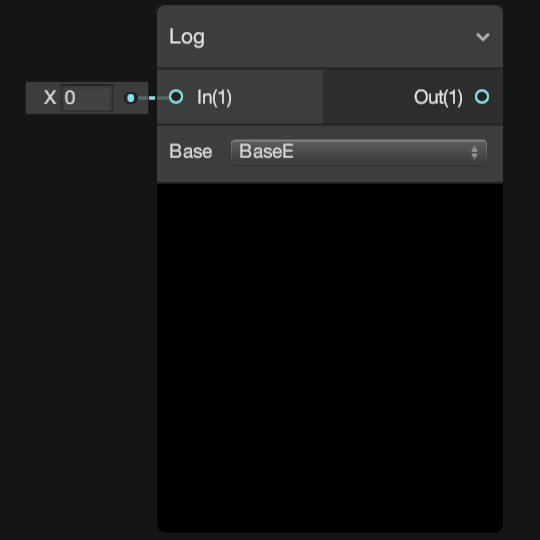Returns the length of input In. Returns the logarithm of input In.
Modulo Negate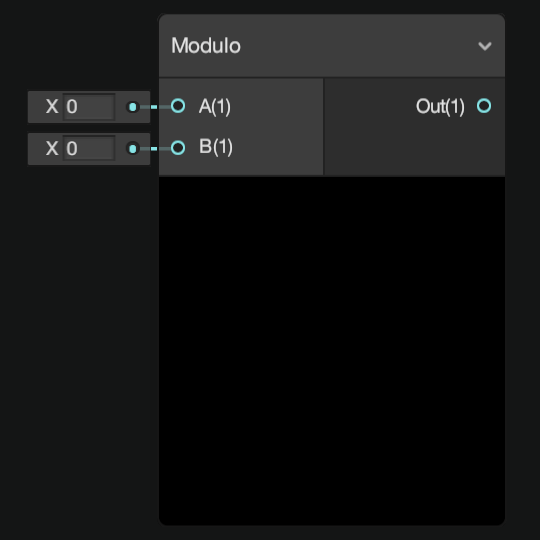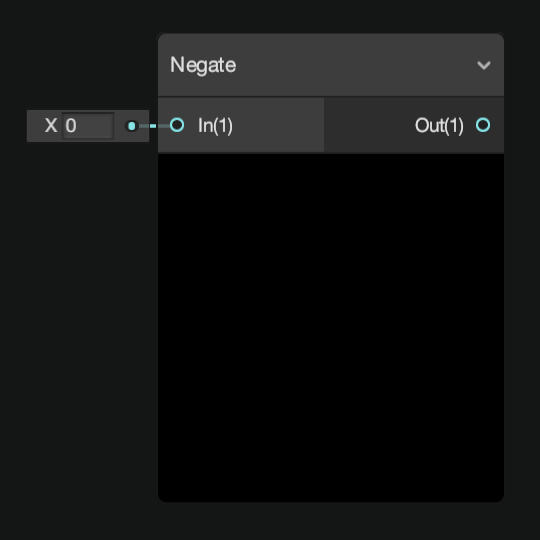Returns the remainder of input A divided by input B. Returns the inverse value of input In.
Normalize Posterize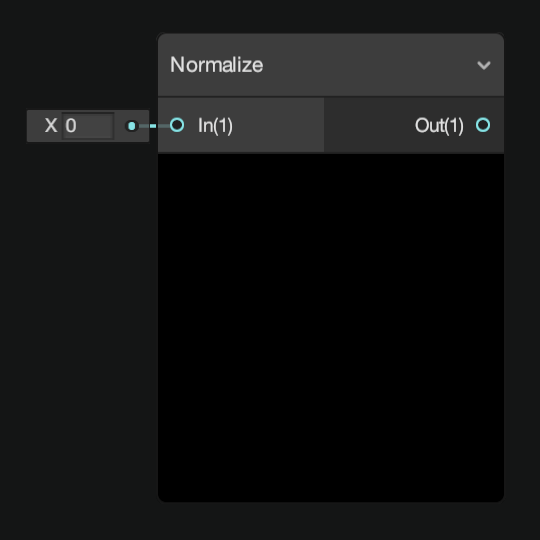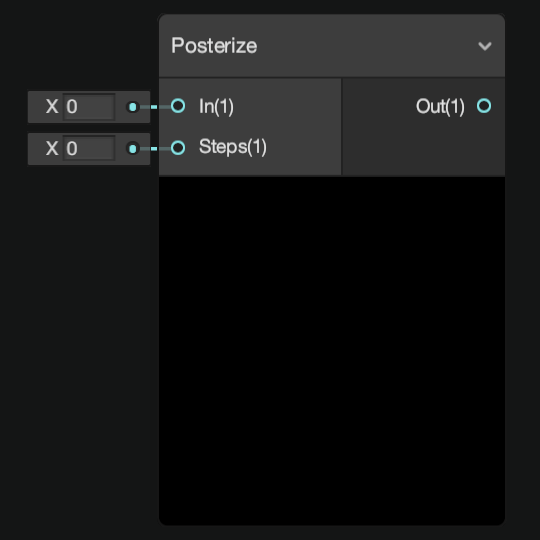Returns the normalized vector of input In. Returns the input In converted into a number of values defined by input Steps.
Reciprocal Reciprocal Square Root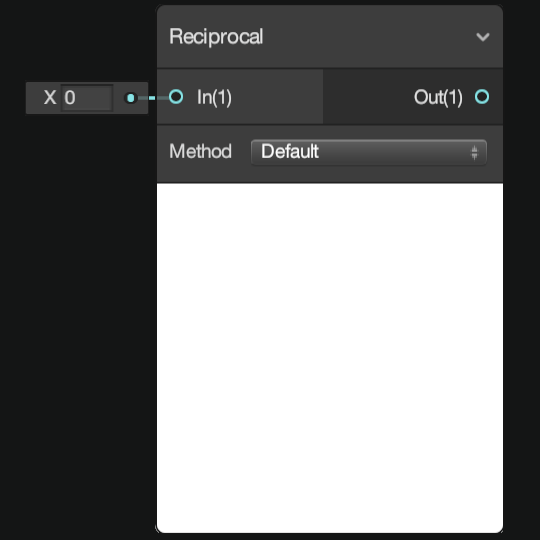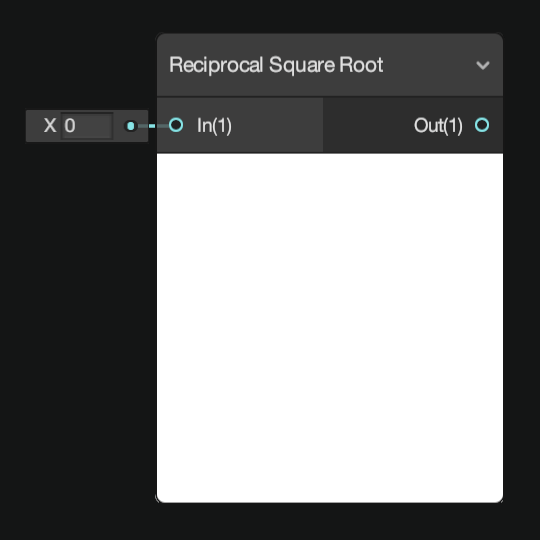Returns the result of 1 divided by input In. Returns the result of 1 divided by the square root of input In.

## Basic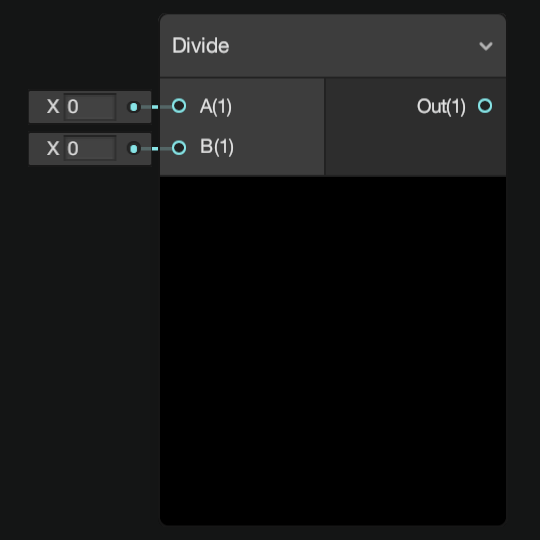Returns the sum of the two input values. Returns the result of input A divided by input B.
Multiply Power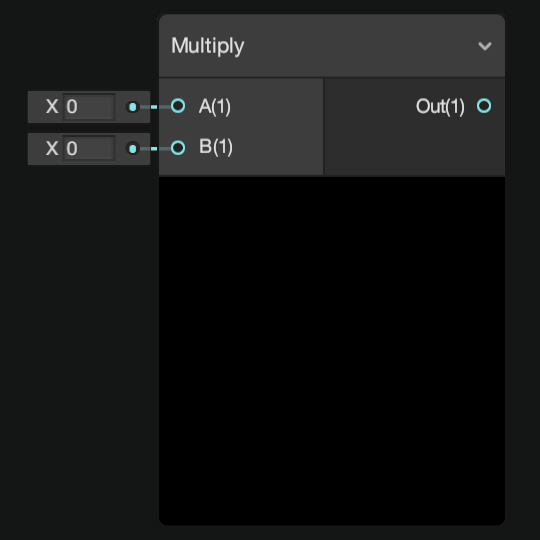Returns the result of input A multiplied by input B. Returns the result of input A to the power of input B.
Square Root Subtract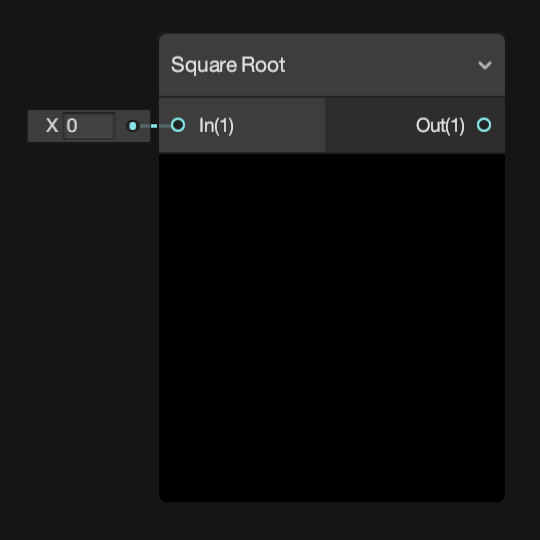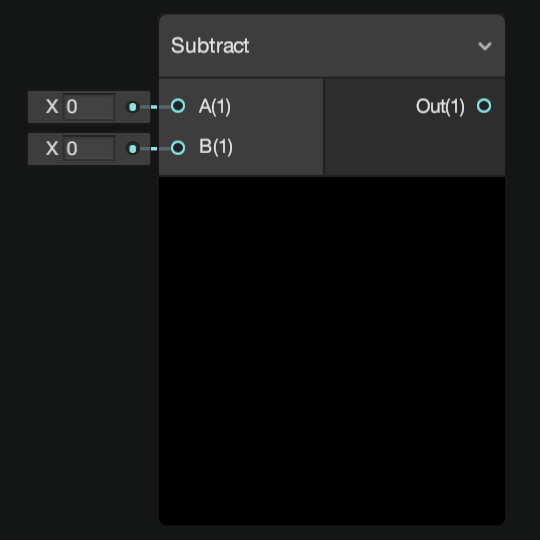Returns the square root of input In. Returns the result of input A minus input B.

## Derivative

DDX DDXY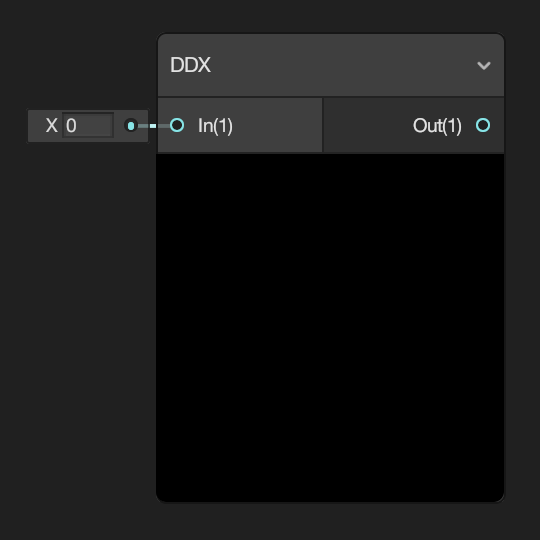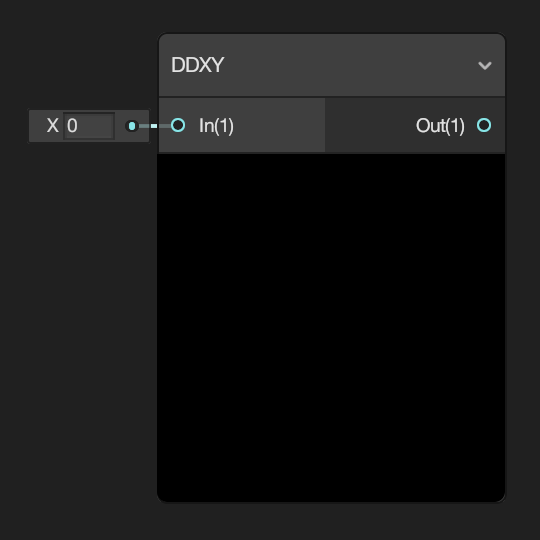Returns the partial derivative with respect to the screen-space x-coordinate. Returns the sum of both partial derivatives.
DDY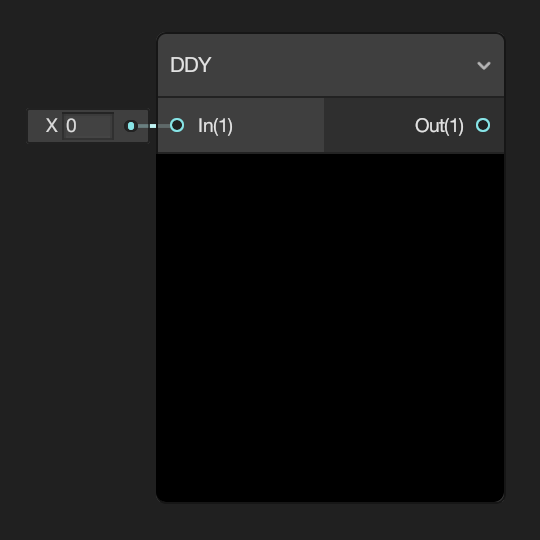Returns the partial derivative with respect to the screen-space y-coordinate.

## Interpolation

Inverse Lerp Lerp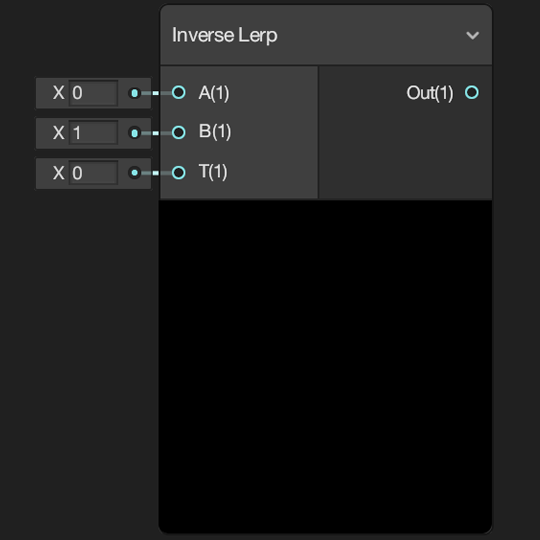Returns the parameter that produces the interpolant specified by input T within the range of input A to input B. Returns the result of linearly interpolating between input A and input B by input T.
Smoothstep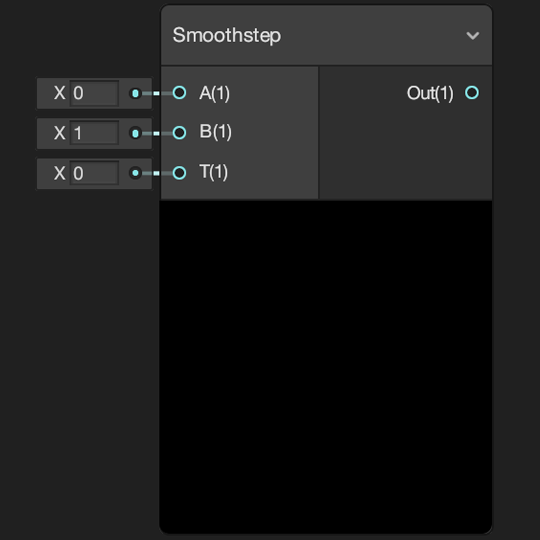Returns the result of a smooth Hermite interpolation between 0 and 1, if input In is between inputs Edge1 and Edge2.

## Matrix

Matrix Construction Matrix Determinant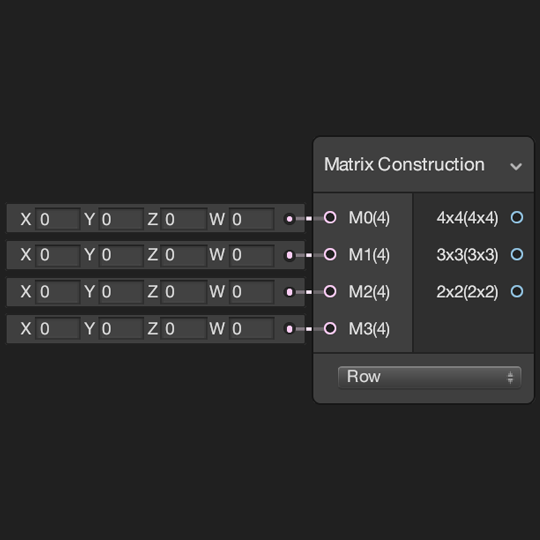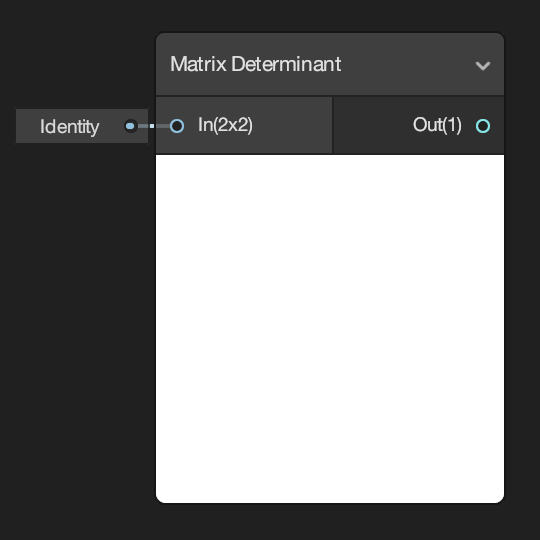Constructs square matrices from the four input vectors M0, M1, M2 and M3. Returns the determinant of the matrix defined by input In.
Matrix Split Matrix Transpose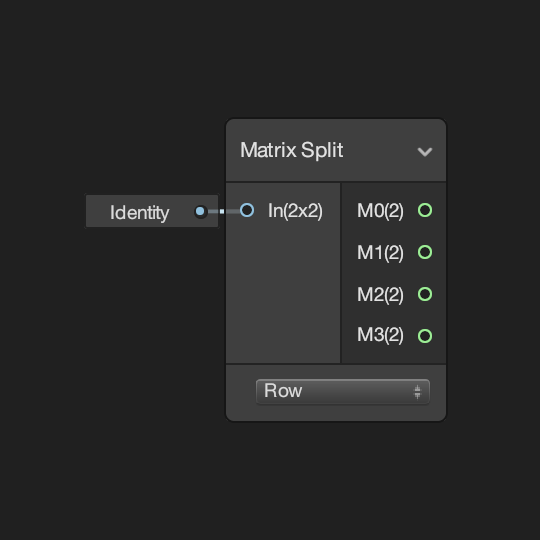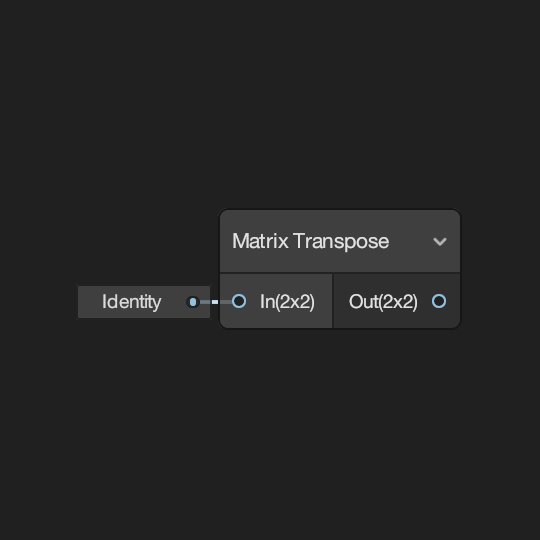Splits a square matrix defined by input In into vectors. Returns the transposed value of the matrix defined by input In.

## Range

Clamp Fraction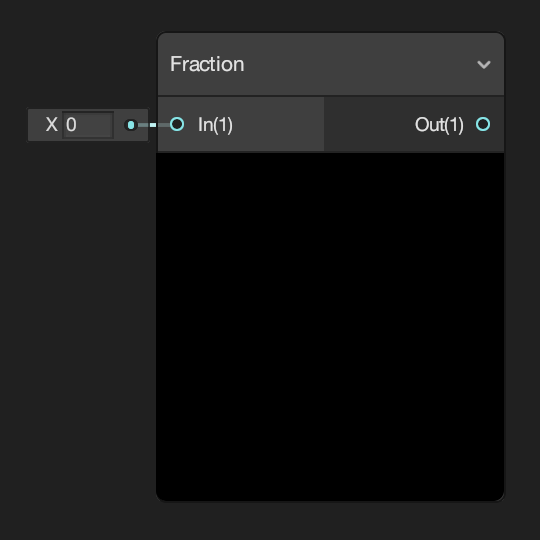Returns the input In clamped between the minimum and maximum values defined by inputs Min and Max respectively. Returns the fractional (or decimal) part of input In; which is greater than or equal to 0 and less than 1.
Maximum Minimum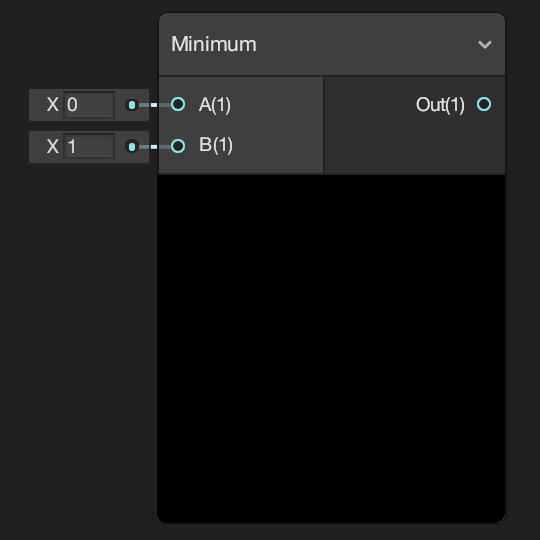Returns the largest of the two inputs values A and B. Returns the smallest of the two inputs values A and B.
One Minus Random Range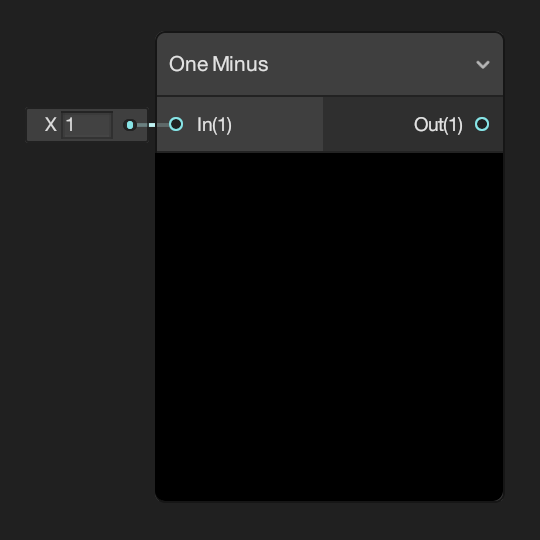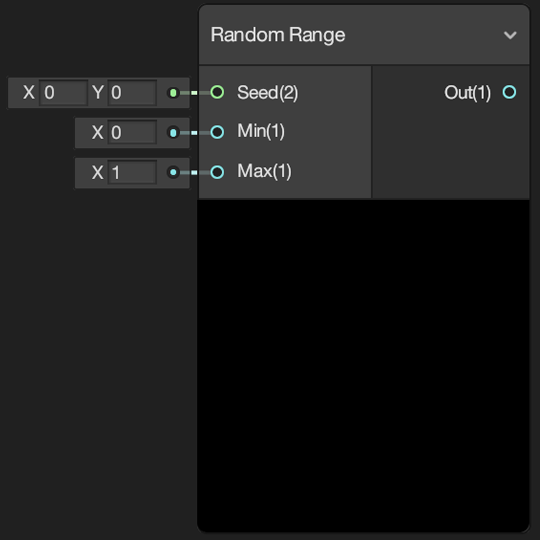Returns the result of input In subtracted from 1. Returns a pseudo-random number that is between the minimum and maximum values defined by inputs Min and Max.
Remap Saturate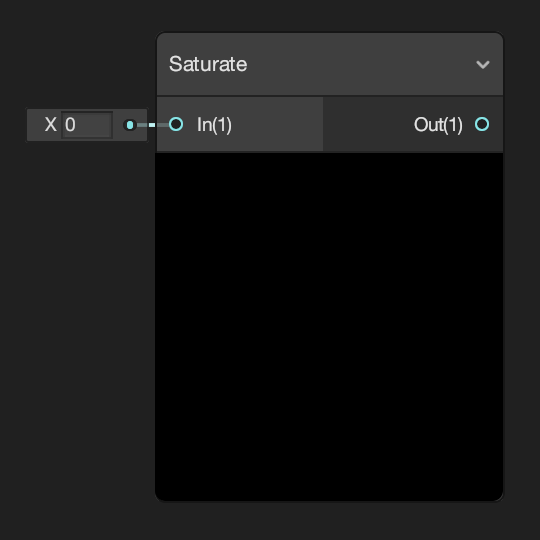Remaps the value of input In from between the values of input Out Min Max to between the values of input In Min Max. Returns the value of input In clamped between 0 and 1.

## Round

Ceiling Floor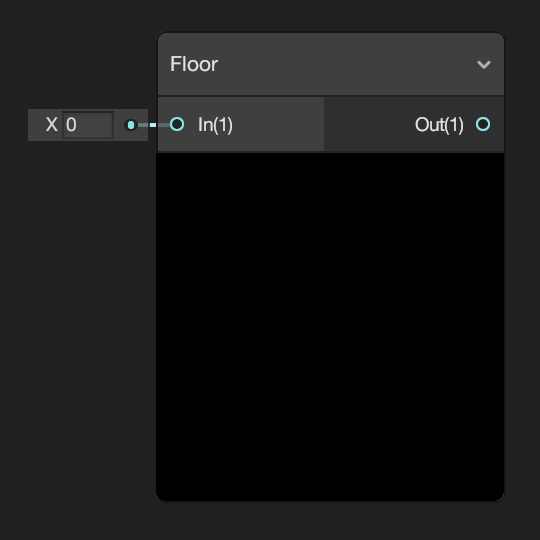Returns the smallest integer value, or whole number, that is greater than or equal to the value of input In. Returns the largest integer value, or whole number, that is less than or equal to the value of input In.
Round Sign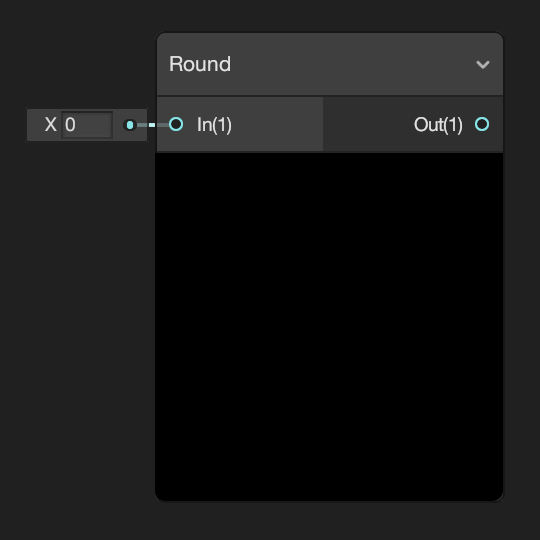Returns the value of input In rounded to the nearest integer, or whole number. Returns -1 if the value of input In is less than zero, 0 if equal to zero and 1 if greater than zero.
Step Truncate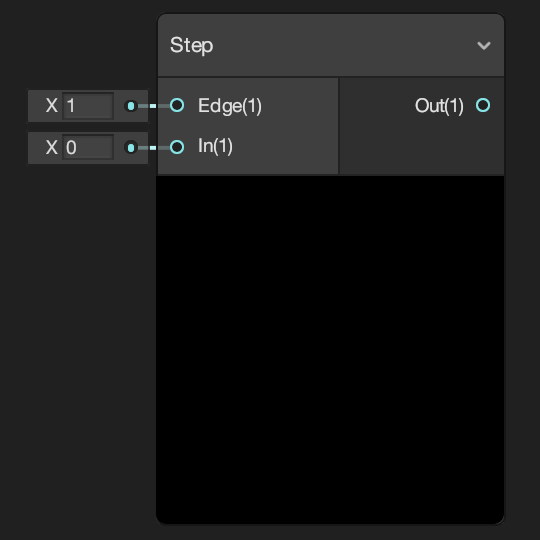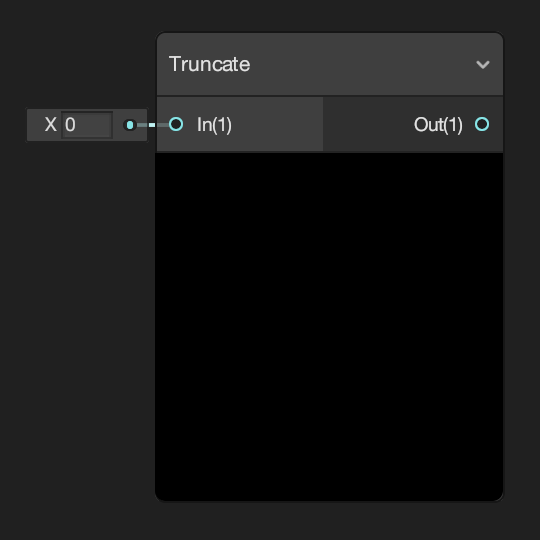Returns 1 if the value of input In is greater than or equal to the value of input Edge, otherwise returns 0. Returns the integer, or whole number, component of the value of input In.

## Trigonometry

Arccosine Arcsine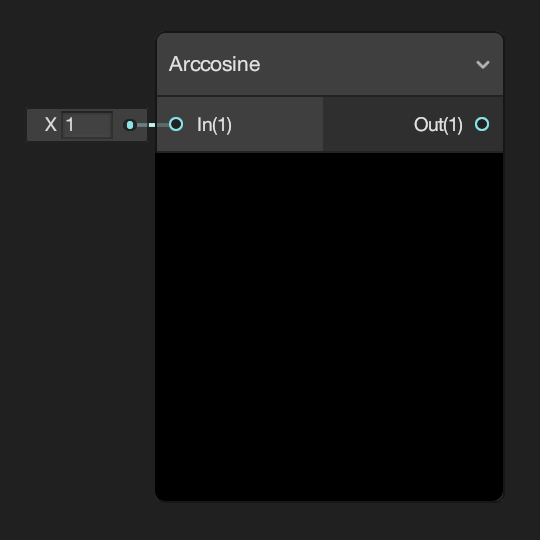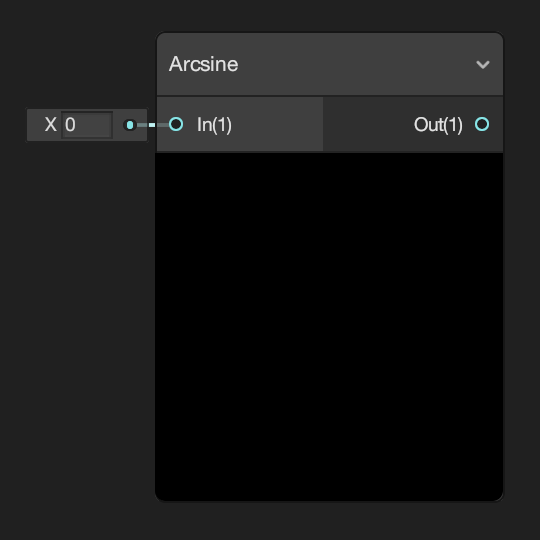Returns the arccosine of each component the input In as a vector of equal length. Returns the arcsine of each component the input In as a vector of equal length.
Arctangent Arctangent2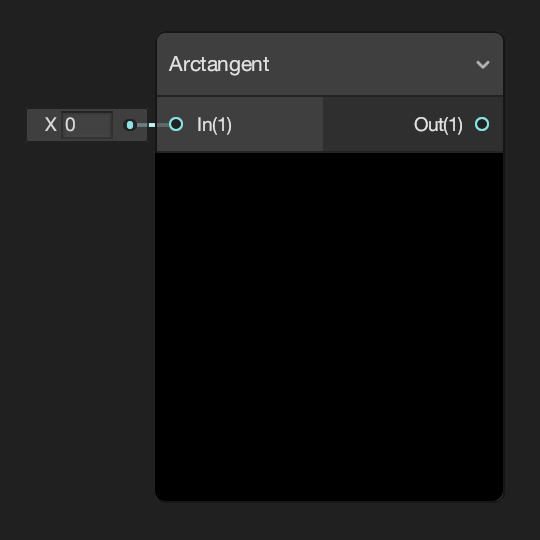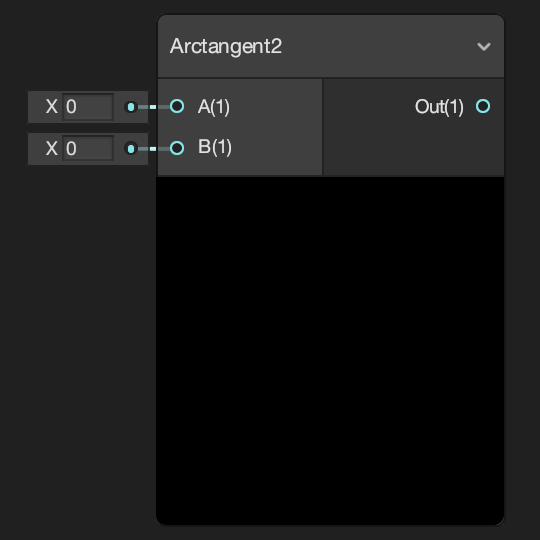Returns the arctangent of the value of input In. Each component should be within the range of -Pi/2 to Pi/2. Returns the arctangent of the values of both input A and input B.Returns the cosine of the value of input In. Returns the value of input In converted from degrees to radians.
Hyperbolic Cosine Hyperbolic Sine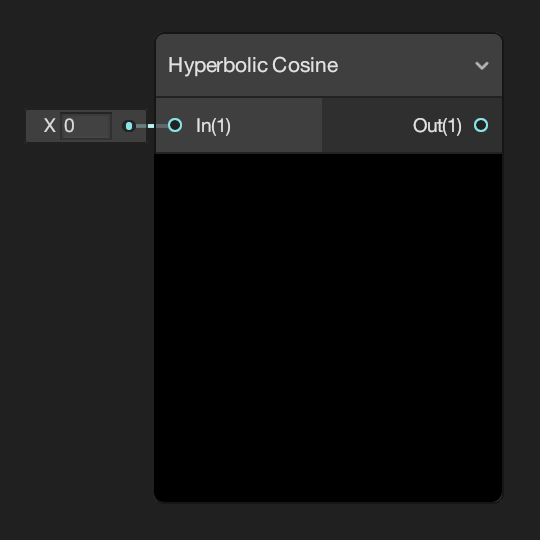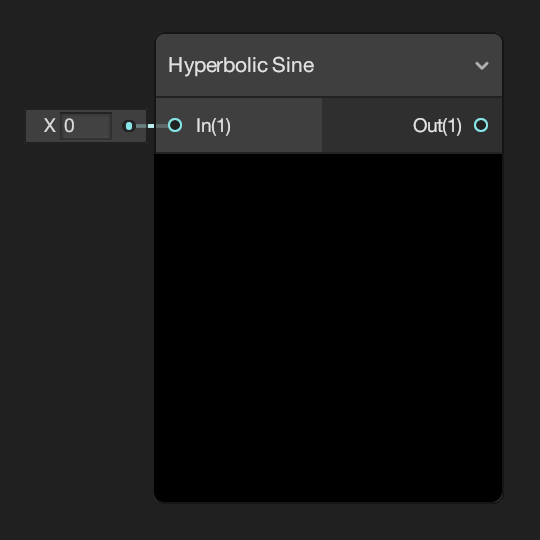Returns the hyperbolic cosine of input In. Returns the hyperbolic sine of input In.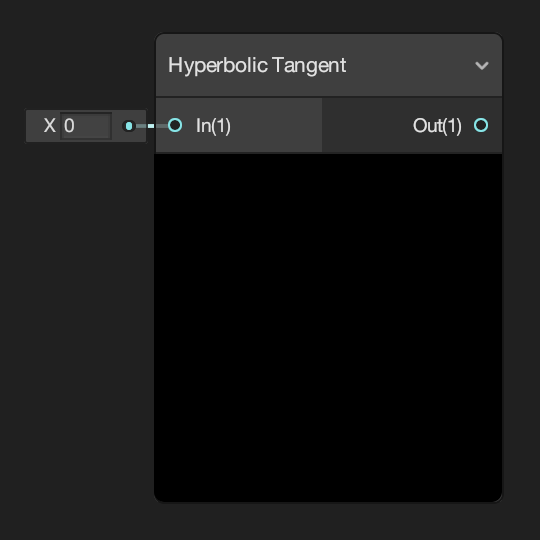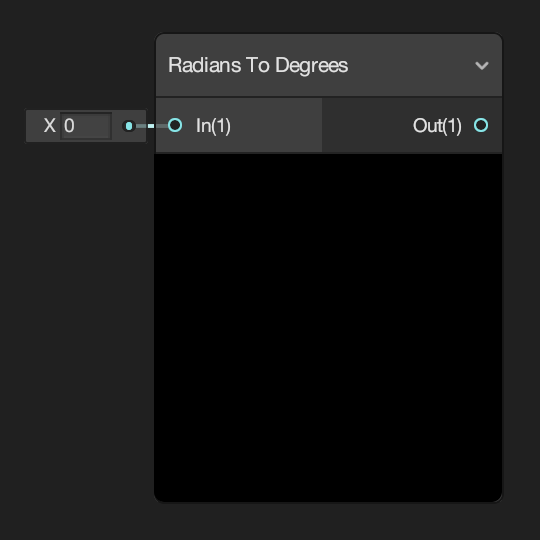Returns the hyperbolic tangent of input In. Returns the value of input In converted from radians to degrees.
Sine Tangent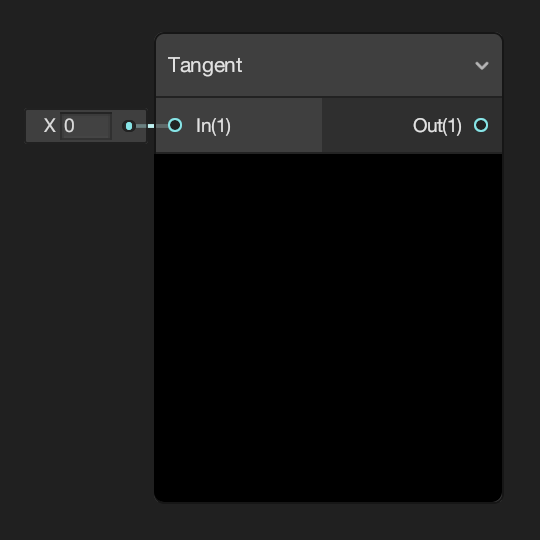Returns the sine of the value of input In. Returns the tangent of the value of input In.

## Vector

Cross Product Distance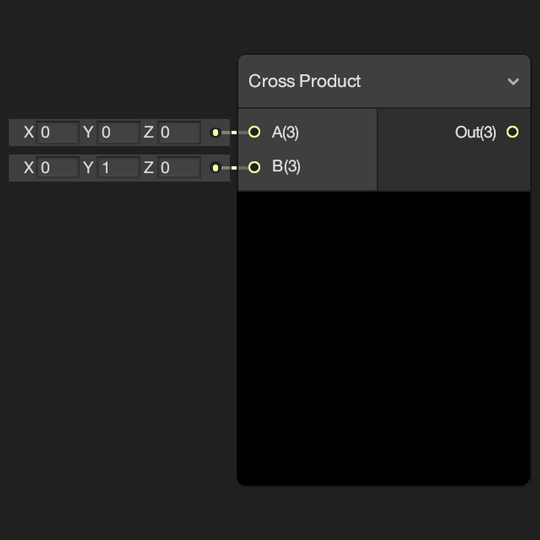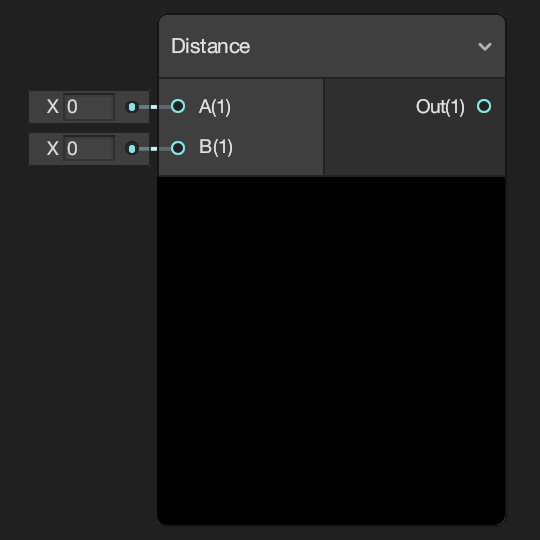Returns the cross product of the values of the inputs A and B. Returns the Euclidean distance between the values of the inputs A and B.
Dot Product Fresnel Effect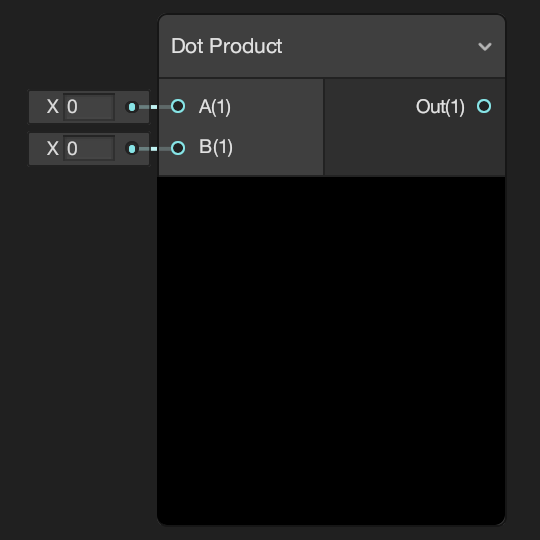Returns the dot product, or scalar product, of the values of the inputs A and B. Fresnel Effect is the effect of differing reflectance on a surface depending on viewing angle, where as you approach the grazing angle more light is reflected.
Projection Reflection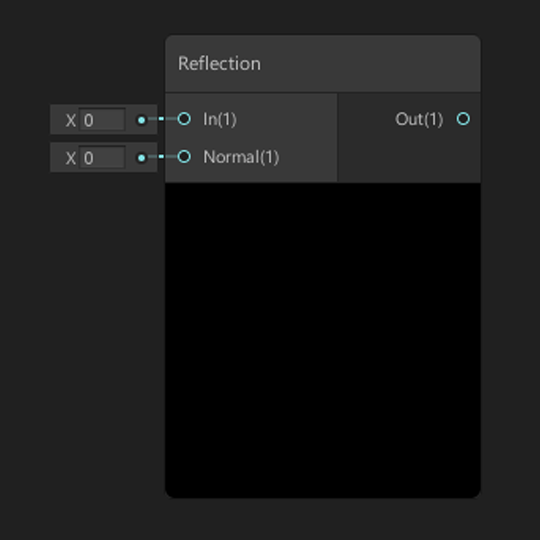Returns the result of projecting the value of input A onto a straight line parallel to the value of input B. Returns a reflection vector using input In and a surface normal Normal.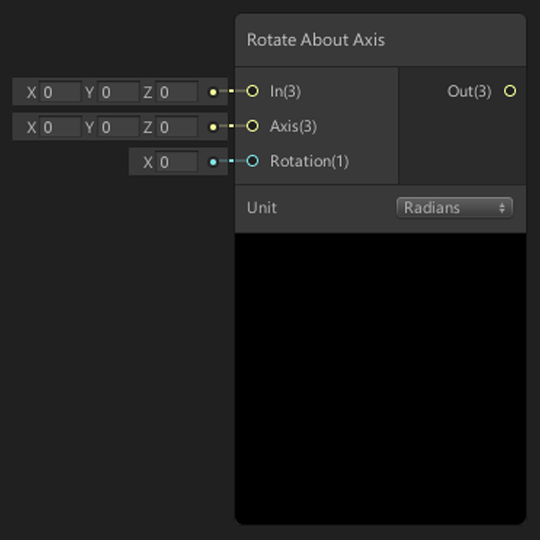Returns the result of the projection of the value of input A onto the plane orthogonal, or perpendicular, to the value of input B. Rotates the input vector In around the axis Axis by the value of Rotation.
Projection RejectionReturns the result of projecting the value of input A onto a straight line parallel to the value of input B. Returns the result of the projection of the value of input A onto the plane orthogonal, or perpendicular, to the value of input B.